<![CDATA[Proceedings of the Edinburgh Mathematical Society]]>https://www.cambridge.org/core/journals/proceedings-of-the-edinburgh-mathematical-society/latest-issueProceedings of the Edinburgh Mathematical Society0013091514643839Cambridge University Press<![CDATA[Virtually spinning hyperbolic manifolds]]>

We give a new proof of a result of Sullivan [Hyperbolic geometry and homeomorphisms, in Geometric topology (ed. J. C. Cantrell), pp. 543–555 (Academic Press, New York, 1979)] establishing that all finite volume hyperbolic n-manifolds have a finite cover admitting a spin structure. In addition, in all dimensions greater than or equal to 5, we give the first examples of finite-volume hyperbolic n-manifolds that do not admit a spin structure.

]]>
https://dx.doi.org/10.1017/S0013091519000324?rft_dat=source%3Ddrssdoi:10.1017/S0013091519000324Virtually spinning hyperbolic manifolds30531310.1017/S0013091519000324https://dx.doi.org/10.1017/S0013091519000324?rft_dat=source%3Ddrss2019-12-05Proceedings of the Edinburgh Mathematical Society2019-12-05Long, D. D.Reid, A. W.
<![CDATA[Some remarks on the volume of log varieties]]>

In this note, using methods introduced by Hacon et al. [‘Boundedness of varieties of log general type’, Proceedings of Symposia in Pure Mathematics, Volume 97 (American Mathematical Society, Providence, RI, 2018) 309–348], we study the accumulation points of volumes of varieties of log general type. First, we show that if the set of boundary coefficients Λ satisfies the descending chain condition (DCC), is closed under limits and contains 1, then the corresponding set of volumes satisfies the DCC and is closed under limits. Then, we consider the case of ε-log canonical varieties, for 0 < ε < 1. In this situation, we prove that if Λ is finite, then the corresponding set of volumes is discrete.

]]>
https://dx.doi.org/10.1017/S0013091519000397?rft_dat=source%3Ddrssdoi:10.1017/S0013091519000397Some remarks on the volume of log varieties31432210.1017/S0013091519000397https://dx.doi.org/10.1017/S0013091519000397?rft_dat=source%3Ddrss2019-12-18Proceedings of the Edinburgh Mathematical Society2019-12-18Filipazzi, Stefano
<![CDATA[Freudenthal's theorem and spherical classes in H*QS0]]>

This note is on spherical classes in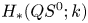when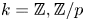, with a special focus on the case of p=2 related to the Curtis conjecture. We apply Freudenthal's theorem to prove a vanishing result for the unstable Hurewicz image of elements in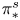that factor through certain finite spectra. After either p-localization or p-completion, this immediately implies that elements of well-known infinite families in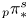, such as Mahowaldean families, map trivially under the unstable Hurewicz homomorphism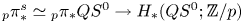. We also observe that the image of the submodule of decomposable elements under the integral unstable Hurewicz homomorphism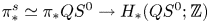is given by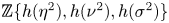. We apply the latter to completely determine spherical classes in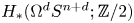for certain values of n>0 and d>0; this verifies Eccles' conjecture on spherical classes in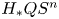, n>0, on finite loop spaces associated with spheres.

]]>
<![CDATA[d-Auslander–Reiten sequences in subcategories]]>

Let Φ be a finite-dimensional algebra over a field k. Kleiner described the Auslander–Reiten sequences in a precovering extension closed subcategory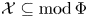. If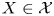is an indecomposable such that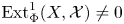and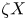is the unique indecomposable direct summand of the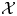-cover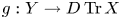such that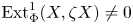, then there is an Auslander–Reiten sequence inof the form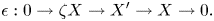Moreover, when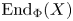modulo the morphisms factoring through a projective is a division ring, Kleiner proved that each non-split short exact sequence of the form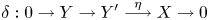is such that η is right almost split in, and the pushout of δ along g gives an Auslander–Reiten sequence in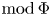ending at X.

In this paper, we give higher-dimensional generalizations of this. Let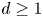be an integer. A d-cluster tilting subcategory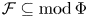plays the role of a higher. Such an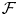is a d-abelian category, where kernels and cokernels are replaced by complexes of d objects and short exact sequences by complexes of d + 2 objects. We give higher versions of the above results for an additive ‘d-extension closed’ subcategoryof.

]]>
<![CDATA[Hypergeometric rational approximations to ζ(4)]]>

We give a new hypergeometric construction of rational approximations to ζ(4), which absorbs the earlier one from 2003 based on Bailey's 9F8 hypergeometric integrals. With the novel ingredients we are able to gain better control of the arithmetic and produce a record irrationality measure for ζ(4).

]]>
<![CDATA[Maximal functions associated with families of homogeneous curves: Lp bounds for P ≤ 2]]>

Let M(u), H(u) be the maximal operator and Hilbert transform along the parabola (t, ut2). For U ⊂ (0, ∞) we consider Lp estimates for the maximal functions sup uU|M(u)f| and sup uU|H(u)f|, when 1 < p ≤ 2. The parabolas can be replaced by more general non-flat homogeneous curves.

]]>
https://dx.doi.org/10.1017/S0013091519000439?rft_dat=source%3Ddrssdoi:10.1017/S0013091519000439Maximal functions associated with families of homogeneous curves: Lp bounds for P ≤ 239841210.1017/S0013091519000439https://dx.doi.org/10.1017/S0013091519000439?rft_dat=source%3Ddrss2020-02-03Proceedings of the Edinburgh Mathematical Society2020-02-03Guo, ShaomingRoos, JorisSeeger, AndreasYung, Po-Lam
<![CDATA[Lyapunov exponents for expansive homeomorphisms]]>

We address the problem of defining Lyapunov exponents for an expansive homeomorphism f on a compact metric space (X, dist) using similar techniques as those developed in Barreira and Silva [Lyapunov exponents for continuous transformations and dimension theory, Discrete Contin. Dynam. Sys.13 (2005), 469–490]; Kifer [Characteristic exponents of dynamical systems in metric spaces, Ergod. Th. Dynam. Sys.3 (1983), 119–127]. Under certain conditions on the topology of the space X where f acts we obtain that there is a metric D defining the topology of X such that the Lyapunov exponents of f are different from zero with respect to D for every point xX. We give an example showing that this may not be true with respect to the original metric dist. But expansiveness of f ensures that Lyapunov exponents do not vanish on a Gδ subset of X with respect to any metric defining the topology of X. We define Lyapunov exponents on compact invariant sets of Peano spaces and prove that if the maximal exponent on the compact set is negative then the compact is an attractor.

]]>
https://dx.doi.org/10.1017/S0013091519000579?rft_dat=source%3Ddrssdoi:10.1017/S0013091519000579Lyapunov exponents for expansive homeomorphisms41342510.1017/S0013091519000579https://dx.doi.org/10.1017/S0013091519000579?rft_dat=source%3Ddrss2020-02-10Proceedings of the Edinburgh Mathematical Society2020-02-10Pacifico, M. J.Vieitez, J. L.
<![CDATA[Enumerating isoclinism classes of semi-extraspecial groups]]>

We enumerate the number of isoclinism classes of semi-extraspecial p-groups with derived subgroup of order p2. To do this, we enumerate GL (2, p)-orbits of sets of irreducible, monic polynomials in 𝔽p[x]. Along the way, we also provide a new construction of an infinite family of semi-extraspecial groups as central quotients of Heisenberg groups over local algebras.

]]>
https://dx.doi.org/10.1017/S0013091519000531?rft_dat=source%3Ddrssdoi:10.1017/S0013091519000531Enumerating isoclinism classes of semi-extraspecial groups42644210.1017/S0013091519000531https://dx.doi.org/10.1017/S0013091519000531?rft_dat=source%3Ddrss2020-02-24Proceedings of the Edinburgh Mathematical Society2020-02-24Lewis, Mark L.Maglione, Joshua
<![CDATA[A preferential attachment process approaching the Rado graph]]>

We consider a simple preferential attachment graph process, which begins with a finite graph and in which a new (t + 1)st vertex is added at each subsequent time step t that is connected to each previous vertex ut with probability du(t)/t, where du(t) is the degree of u at time t. We analyse the graph obtained as the infinite limit of this process, and we show that, as long as the initial finite graph is neither edgeless nor complete, with probability 1 the outcome will be a copy of the Rado graph augmented with a finite number of either isolated or universal vertices.

]]>
<![CDATA[Mixed Bruce–Roberts numbers]]>

We extend the notions of μ*-sequences and Tjurina numbers of functions to the framework of Bruce–Roberts numbers, that is, to pairs formed by the germ at 0 of a complex analytic variety X ⊆ ℂn and a finitely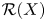-determined analytic function germ f : (ℂn, 0) → (ℂ, 0). We analyze some fundamental properties of these numbers.

]]>
https://dx.doi.org/10.1017/S0013091519000543?rft_dat=source%3Ddrssdoi:10.1017/S0013091519000543Mixed Bruce–Roberts numbers45647410.1017/S0013091519000543https://dx.doi.org/10.1017/S0013091519000543?rft_dat=source%3Ddrss2020-02-27Proceedings of the Edinburgh Mathematical Society2020-02-27Bivià-Ausina, CarlesRuas, Maria Aparecida Soares
<![CDATA[Delta- and Daugavet points in Banach spaces]]>

A Δ-point x of a Banach space is a norm-one element that is arbitrarily close to convex combinations of elements in the unit ball that are almost at distance 2 from x. If, in addition, every point in the unit ball is arbitrarily close to such convex combinations, x is a Daugavet point. A Banach space X has the Daugavet property if and only if every norm-one element is a Daugavet point. We show that Δ- and Daugavet points are the same in L1-spaces, in L1-preduals, as well as in a big class of Müntz spaces. We also provide an example of a Banach space where all points on the unit sphere are Δ-points, but none of them are Daugavet points. We also study the property that the unit ball is the closed convex hull of its Δ-points. This gives rise to a new diameter-two property that we call the convex diametral diameter-two property. We show that all C(K) spaces, K infinite compact Hausdorff, as well as all Müntz spaces have this property. Moreover, we show that this property is stable under absolute sums.

]]>
https://dx.doi.org/10.1017/S0013091519000567?rft_dat=source%3Ddrssdoi:10.1017/S0013091519000567Delta- and Daugavet points in Banach spaces47549610.1017/S0013091519000567https://dx.doi.org/10.1017/S0013091519000567?rft_dat=source%3Ddrss2020-02-27Proceedings of the Edinburgh Mathematical Society2020-02-27Abrahamsen, T. A.Haller, R.Lima, V.Pirk, K.
<![CDATA[Generation of relative commutator subgroups in Chevalley groups. II]]>

In the present paper, which is a direct sequel of our paper  joint with Roozbeh Hazrat, we prove an unrelativized version of the standard commutator formula in the setting of Chevalley groups. Namely, let Φ be a reduced irreducible root system of rank ≥ 2, let R be a commutative ring and let I,J be two ideals of R. We consider subgroups of the Chevalley group G(Φ, R) of type Φ over R. The unrelativized elementary subgroup E(Φ, I) of level I is generated (as a group) by the elementary unipotents xα(ξ), α ∈ Φ, ξ ∈ I, of level I. Obviously, in general, E(Φ, I) has no chance to be normal in E(Φ, R); its normal closure in the absolute elementary subgroup E(Φ, R) is denoted by E(Φ, R, I). The main results of  implied that the commutator [E(Φ, I), E(Φ, J)] is in fact normal in E(Φ, R). In the present paper we prove an unexpected result, that in fact [E(Φ, I), E(Φ, J)] = [E(Φ, R, I), E(Φ, R, J)]. It follows that the standard commutator formula also holds in the unrelativized form, namely [E(Φ, I), C(Φ, R, J)] = [E(Φ, I), E(Φ, J)], where C(Φ, R, I) is the full congruence subgroup of level I. In particular, E(Φ, I) is normal in C(Φ, R, I).

]]>
https://dx.doi.org/10.1017/S0013091519000555?rft_dat=source%3Ddrssdoi:10.1017/S0013091519000555Generation of relative commutator subgroups in Chevalley groups. II49751110.1017/S0013091519000555https://dx.doi.org/10.1017/S0013091519000555?rft_dat=source%3Ddrss2020-03-02Proceedings of the Edinburgh Mathematical Society2020-03-02Vavilov, NikolaiZhang, Zuhong
<![CDATA[Principal co-Higgs bundles on ℙ1]]>

For complex connected, reductive, affine, algebraic groups G, we give a Lie-theoretic characterization of the semistability of principal G-co-Higgs bundles on the complex projective line ℙ1 in terms of the simple roots of a Borel subgroup of G. We describe a stratification of the moduli space in terms of the Harder–Narasimhan type of the underlying bundle.

]]>
The aim of this paper is to show the importance of the Steenrod construction of homology theories for the disassembly process in surgery on a generalized n-manifold Xn, in order to produce an element of generalized homology theory, which is basic for calculations. In particular, we show how to construct an element of the nth Steenrod homology group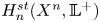, where 𝕃+ is the connected covering spectrum of the periodic surgery spectrum 𝕃, avoiding the use of the geometric splitting procedure, the use of which is standard in surgery on topological manifolds.